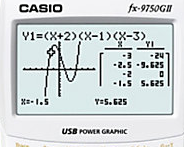## Database Building 101The cost of graphing

time to read 2 min | 368 wordsSo far we looked into how we can store the nodes and the edges, and we explored some interesting data structures inside Voron. Now, let’s see how we can traverse the graph.

So getting the node is pretty easy, and remember that to access the table by ID gives us an O(1) cost.

Now, what about finding all the edges from a node?

This is a lot heftier, but let’s try to break it into individual pieces. First, we find the tree holding all the edges of that particular type, then we access (by the ID of the source node) the fixed size tree that holds all the connected nodes and the edge data ID.

Finally, we access the edges data (if it exists) to get the data about the edge itself, after which, we return it all to the caller.

Unlike the previous method, here we can’t claim to have O(1) costs. Instead, our costs are composed of:

1. O(logN) – where N is the number of edges types (typically, very few), to search for the right edges tree.
2. O(logN) – where N is the number of source nodes (typically, very large, but the fixed size trees are very good at being fast, and they don’t have to do much).
3. O(N) – where N is the number of connection between the source node and the destination node.

I’m excluding the cost of loading the edge data, since this is an O(1) operation and is directly relevant to the iteration over all nodes connected to the source node.

Ideally, we can find a way to turn the 2nd operation into an O(1) cost, but that should be more than good enough for the moment.

Now, this just gives us traversal of the nodes, but going from here to Breadth First Search / Depth First Search and doing them well is fairly trivial, although there are a few things that we'll need to talk about, but that would serve as a separate post.